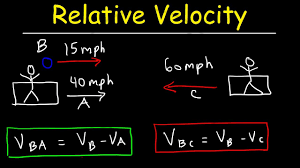Share

# Relative Velocity

## Relative velocity• When you are travelling in a car or bus or train, you see the trees, buildings and many other things outside going backwards. But are they really going backwards? No, you know it pretty well that it’s your vehicle that is moving while the trees are stationary on the ground. But then why do the trees appear to be moving backwards? Also the co-passengers with you who are moving appear stationary to you despite moving.

• It’s because in your frame both you and your co-passengers are moving together. Which means there is no relative velocity between you and the passengers. Whereas the trees are stationary while you are moving. Therefore trees are moving at some relative velocity with respect to you and the other passenger. And that relative velocity is the difference of velocities between you and the tree.

The relative velocity is the velocity of an object or observer B in the rest frame of another object or the observer A. The general formula of velocity is:

Velocity of B relative to A is = barv_b-barv_a

This is the only formula that describes the concept of relative velocity. When two objects are moving in the same direction, then
bar v_(ab)=bar v_a+bar v_b
When two objects are moving in the opposite direction, then
bar v_(ab)=bar v_a-bar v_b

Lets us understand the concept of relative velocity with this example.Consider two trains moving with same speed and in the same direction. Even if both the trains are in motion with respect to buildings, trees along the two sides of the track, yet to the observer of the train, the other train does not seem to be moving at all. the velocity of the other train appears to be zero.

Suppose you are in a car moving at 50 mph. The 50 mph is your relative velocity as compared to the surface of the earth. At the same time if I am sitting next to you your relative velocity compared to me is zero. If we were on a bus and you walked forward at 1 mph, your relative velocity on the earth would be 51 mph and your relative velocity compared to me would be 1 mph. Relative velocity is simply any objects speed compared to any other object regardless of its speed.

### Shaalaa.com

Straight line motion Part 19 (Relative velocity) [00:09:43]
S
1
0%

S